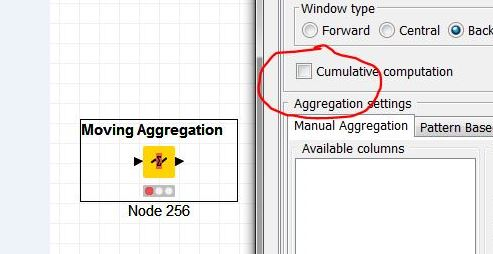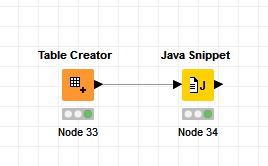# Dynamic Formula

Hey everyone,
I’ve been trying to duplicate an excel formula in Knime but I don’t know how.
Here’s my problem:
My input table is similar to this one

Month Quantity Data Calculated quantity
1 A X A+X
2 A+X Y A+X+Y
3 A+X+Y Z A+X+Y+Z

The calculated quantity changes depending on the month. It always adds the last month quantity.

How can I have the same result in Knime?

Thank you

You can use the Moving Aggregation node, with the option cumulative computation.
cum_computation.knwf (7.8 KB)2 Likes

Hi @HansS , The quantity column should be apdated when the calculated quantity is added.
So my input table is as follows:

Month Quantity Data Calculated quantity
1 A X A+X
2 Y A+X+Y

For month 2, quantity will be = Calculated quantity and so on…

@newuser01

Sorry for the misunderstanding. It will be helpfull if you provide an example table (take 5 rows) of your input table AND your output table.

Okay, so here’s my input table:

Month Quantity Quantity used Data Calculated quantity
1 2 2 1 2+1
2 3 0
4 4 4
5 3 2

When the “calculed quantity” is updated , its value is added to the “quantity” to calculate again the "calculated quantity for the next month:

Month Quantity Quantity used Data Calculated quantity
1 2 2 1 2+1
2 3 2+1+3 0 2+1+3+0
4 4 4
5 3 2

And so on, untill all the cells are calculated:

Month Quantity Quantity used Data Calculated quantity
1 2 2 1 2+1
2 3 2+1+3 0 2+1+3+0
4 4 2+1+3+0+4 4 2+1+3+0+4+4
5 3 2

The final output is:

Month Quantity Quantity used Data Calculated quantity
1 2 2 1 2+1
2 3 2+1+3 0 2+1+3+0
4 4 2+1+3+0+4 4 2+1+3+0+4+4
5 3 2+1+3+0+4+4+3 2 2+1+3+0+4+4+3+2

It’s like a loop.

Use Moving Aggregation node first for Quantity used column based on a quantity column (preliminary calc).
Lag Column node to Data and use
Math Formula Multi columns to calculate Quantity Used (add lagged column) and Calculate quantity using Data column and Quantity used.

@newuser01

Ah, that’s a little bit more complex but I think this what your are looking for.

see the workflow:
cum_computation_2.knwf (28.0 KB)
gr. Hans

3 Likes

Thank you, it’s working !

Hi @newuser01,

you can also solve it with a java snippet node. Here is an example.Example.knwf (6.3 KB)

Thank you, but i’ve never programmed with Java.
Could you help me with something else as well?

Hi @newuser01,
Please can you explain it with an example shown as a table or if you have the calculation available in a Excel spreadsheet you can also upload this example file.
Thanks

example.xlsx (9.3 KB)

Here’s an example:
The data column is changed manually so that the extra is equal to 0.
But I want to automate this process using Knime.
And at the same time, the other calculations are happening

Hi @morpheus
Did you check the example that I uploaded?

Thanks

Hi @newuser01,
adding an additional column is not the problem. But i don’t get the role of the column data, which seems to drive your final outcome. Your explanation and the example is not very helpful.

For your understanding how java scripts has to be defined i added in the attached example the Column “target” in the source table and the calculation for the column “Extra” in the Java snippet. Comparing with the previous example you can see how the java snippet works.

Example.knwf (7.1 KB)

1 Like

Hi @morpheus
I wanted to add a new condition on the Extra Column , is this formula correct?

if (out_Extra == null) {out_newquantity = Calc_Quant_Val;}
else {out_newquantity = c_target ;Quant_Used_Val = out_nEWsHIP + c_Quantity;out_newquantity = Quant_Used_Val + c_Data;out_Quantityusednew = Quant_Used_Val;out_calculatedquantitynew = out_newquantity;out_newextra = out_newquantity - c_target;}

If the extra column isn’t null , the data should be updated so that Extra becomes equal to 0.

Hi @newuser01,
what happens in the case that Extra equals null?
Please consider the depencense of the data and therefore the correct assignment (order of statements).
Run the java node and you’ll get the result and see whats happens.

This topic was automatically closed 182 days after the last reply. New replies are no longer allowed.